# W2NER: Unified Named Entity Recognition as Word - Word Relation Classification

## Basic Idea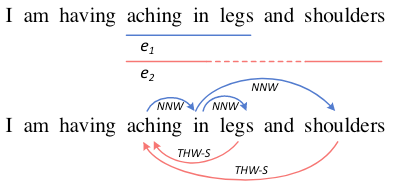## W2NER

### NER as Word-Word Relation Classification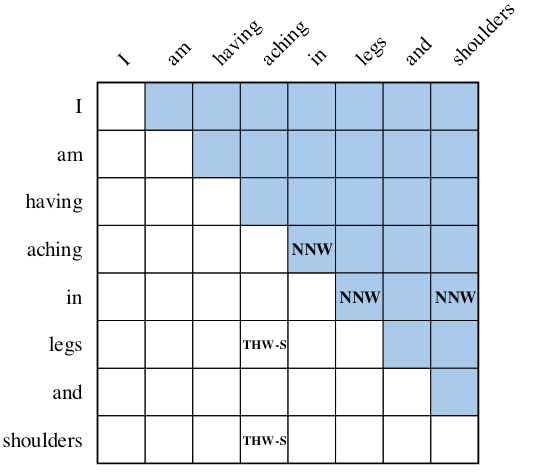• $\text{None}$: 该Token Pair之间不存在任何关系.
• $\text{Next-Neighboring-Word}$: $(x_{i,}x_j)$ 位于一个实体提及中, 且在该实体提及中, 行Token $x_i$ 是列Token $x_j$ 的前一个Token.
• $\text{Tail-Head-Word-}\star$: $(x_{i,}x_j)$ 位于一个实体提及中, 且在该实体提及中, 行Token $x_i$ 是该实体提及的结束Token, 列Token $x_j$ 为该实体提及的起始Token. $\star$ 代表该实体提及的实体类型.

### Unified NER Framework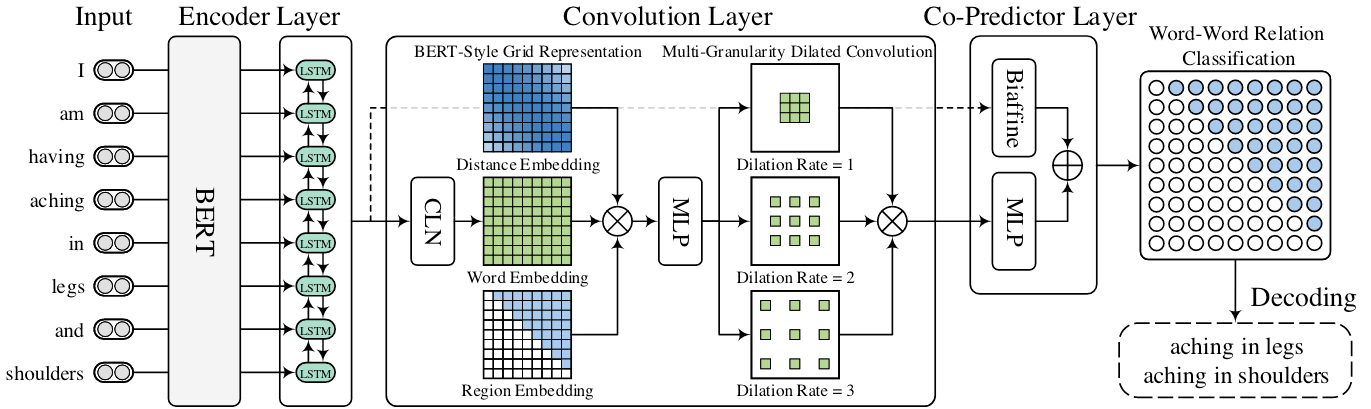#### Encoder Layer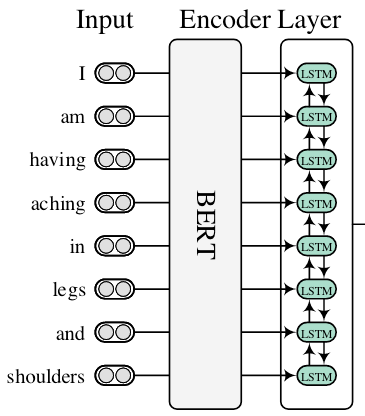#### Convolution Layer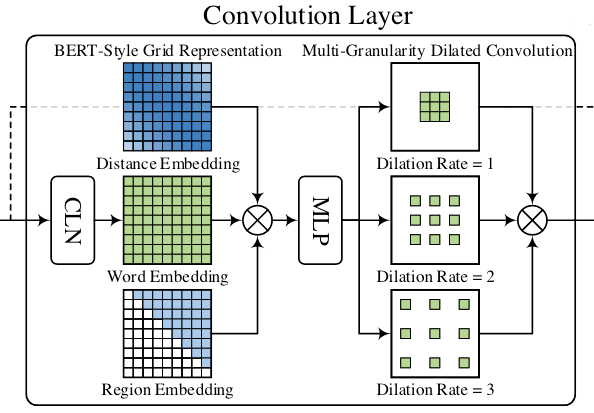##### Conditional Layer Normalization

$$\mathbf{V}_{i j}=\operatorname{CLN}\left(\mathbf{h}_i, \mathbf{h}_j\right)=\gamma_{i j} \odot\left(\frac{\mathbf{h}_j-\mu}{\sigma}\right)+\lambda_{i j}$$

$\mathbf{h}_i$ 将用于生成缩放系数$\gamma_{ij}$ 和平移系数$\lambda_{ij}$:

\begin{aligned} \gamma_{ij} = \mathbf{W}_{\alpha}\mathbf{h}_{i}+ \mathbf{b}_{\alpha}\\ \lambda_{ij} = \mathbf{W}_{\beta}\mathbf{h}_{i}+ \mathbf{b}_{\beta} \end{aligned}

$\mu, \sigma$ 分别是$\mathbf{h}_j$ 中跨元素的均值和标准差:

$$\mu=\frac{1}{d_h} \sum_{k=1}^{d_h} h_{j k}, \quad \sigma=\sqrt{\frac{1}{d_h} \sum_{k=1}^{d_h}\left(h_{j k}-\mu\right)^2}$$

$h_{jk}$ 代表$\mathbf{h}_j$ 的第$k$ 维.

##### BERT-Style Grid Representation Build - Up

• CLN得到的Word Pair表示$\mathbf{V} \in \mathbb{R}^{N \times N \times d_h}$.
• Word Pair之间的相对位置信息记作$\mathbf{E}^d \in \mathbb{R}^{N \times N \times d_{E_d}}$.
• 上下三角的区域Embedding$\mathbf{E}^t \in \mathbb{R}^{N \times N \times d_{E_t}}$.

$$\mathbf{C}=\operatorname{MLP}_1\left(\left[\mathbf{V} ; \mathbf{E}^d ; \mathbf{E}^t\right]\right)$$

##### Multi - Granularity Dilated Convolution

$$\mathbf{Q}^l=\sigma\left(\operatorname{DConv}_l(\mathbf{C})\right)$$

$l$ 为空洞卷积的膨胀系数.

$$\mathbf{Q}= \left[\mathbf{Q}^{1}, \mathbf{Q}^{2}, \mathbf{Q}^{3}\right] \in \mathbb{R}^{N \times N \times 3d_c}$$

#### Co - Predictor Layer

Co - Predictor由双仿射和MLP两个组件同时对前面Encoder Layer的特征$\mathbf{H} \in \mathbb{R}^{N \times d_h}$ 和Convolution Layer特征$\mathbf{Q} \in \mathbb{R}^{N \times N \times 3d_c}$ 做聚合, 得到Logits: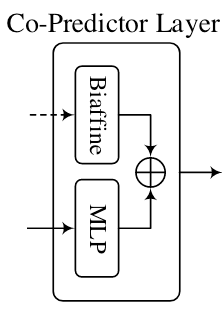##### Biaffine Predictor

Biaffine Predictor的作用对象是Encoder所抽取出的特征, 用两个MLP分别获得Subject和Object对应的表示, 然后再用双仿射获取Logits:

\begin{aligned} \mathbf{s}_i &=\operatorname{MLP}_2\left(\mathbf{h}_i\right) \\ \mathbf{o}_j &=\operatorname{MLP}_3\left(\mathbf{h}_j\right) \\ \mathbf{y}_{i j}^{\prime} &=\mathbf{s}_i^{\top} \mathbf{U} \mathbf{o}_j+\mathbf{W}\left[\mathbf{s}_i ; \mathbf{o}_j\right]+\mathbf{b} \end{aligned}

##### MLP Predictor

MLP的作用对象是前面空洞卷积获得的特征$\mathbf{Q}$, 用于聚合Convolution Layer获得的每个格子中的信息:

$$\mathbf{y}_{i j}^{\prime \prime}=\operatorname{MLP}\left(\mathbf{Q}_{i j}\right)$$

$$\mathbf{y}_{i j}=\operatorname{Softmax}\left(\mathbf{y}_{i j}^{\prime}+\mathbf{y}_{i j}^{\prime \prime}\right)$$

#### Decoding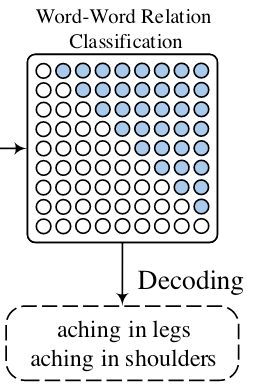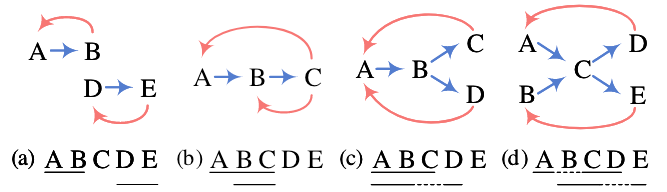• (a): 两个Flat实体. 对于简单的完全Flat的情况, 其实两条路径A -> B, D -> E就可以标注出来, THW在此时仅标注它们的实体类型.
• (b): 两个重叠实体(该情况也是嵌套), 此时单纯用NNW没法解码出两个嵌套实体, 但THW在使得其可以解码出两个嵌套实体ABC, BC.
• (c): 含有重叠的一个Flat实体和一个不连续实体. NNW可以找到A -> B -> CA -> B -> D这两条边, 在THW的辅助下解码出实体ABCABD.
• (d): 最为复杂的情况, 两个部分重叠且不连续的实体. 同样是通过NNW无法单独解码出, 但在THW辅助下可解码出.

#### Learning

$$\mathcal{L}=-\frac{1}{N^2} \sum_{i=1}^N \sum_{j=1}^N \sum_{r=1}^{|\mathcal{R}|} \hat{\mathbf{y}}_{i j}^r \log \mathbf{y}_{i j}^r$$

## Experiments

### Datasets

• Flat: CoNLL - 2003, OntoNotes 5.0(English), OntoNotes 4.0, MSRA, Weibo, Resume.
• Overlapped: ACE2004, ACE2005, GENIA.
• Discontinuous: 三个英文数据集CADEC, ShARe13, ShARe14, 两个中文数据集ACE2004, ACE2005.

### Flat NER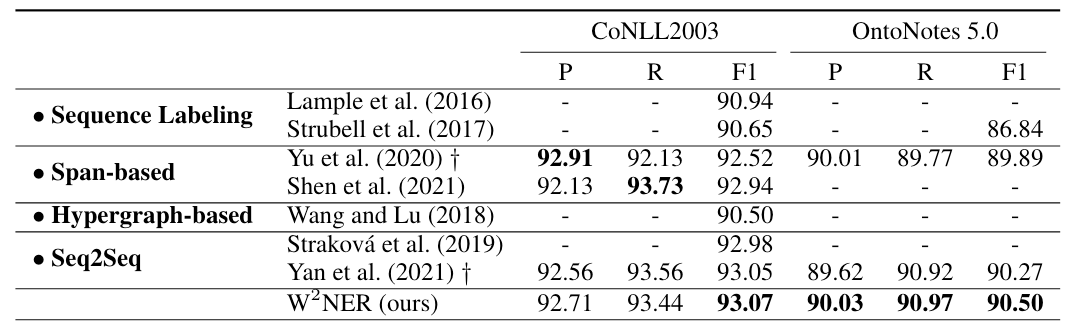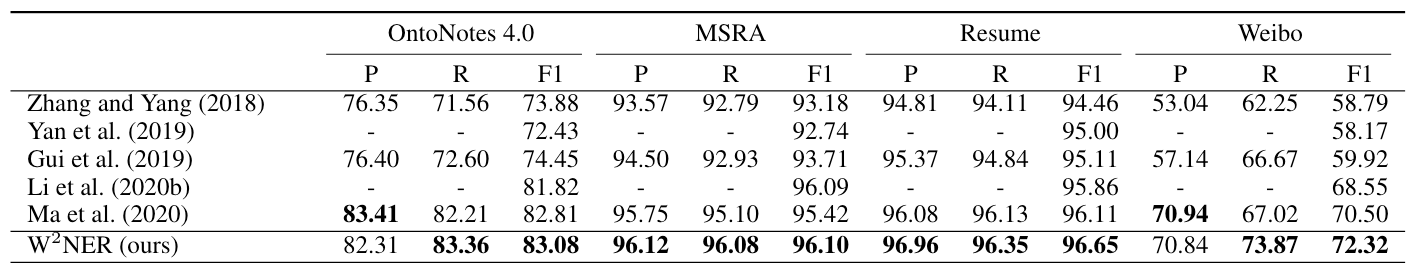### Overlapped NER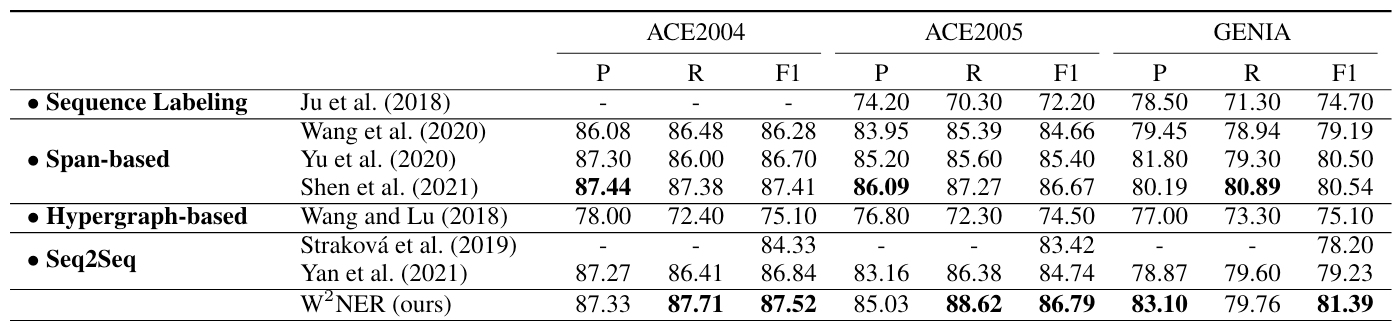### Discontinuous NER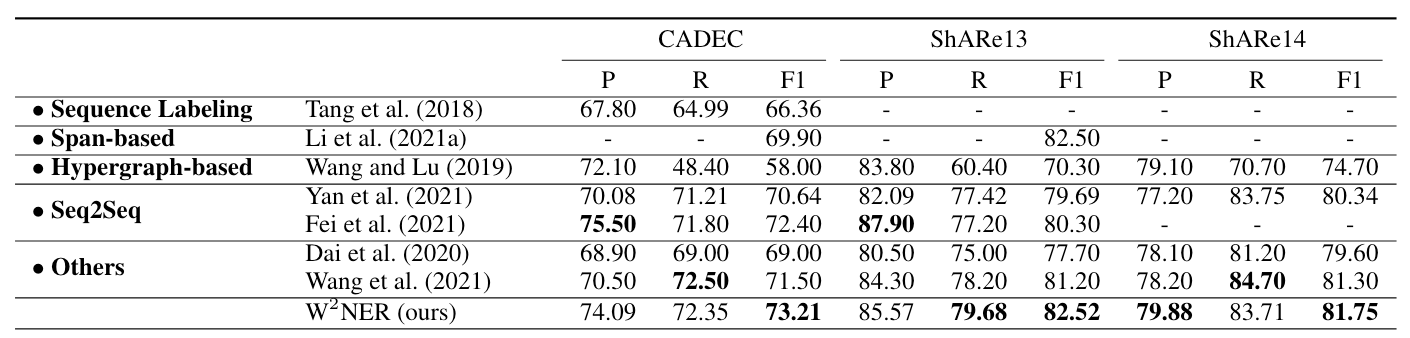W2NER较其他方法拥有很好的性能, 要记得它在其他NER上表现仍然很猛.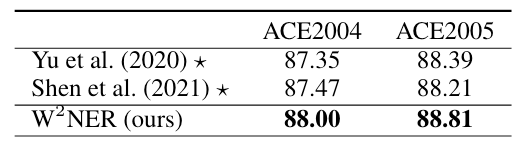Baseline两篇论文并没有在中文数据集上跑过, 这是作者自行使用官方源码得到的结果. 在两个数据集上领先Baseline半个点.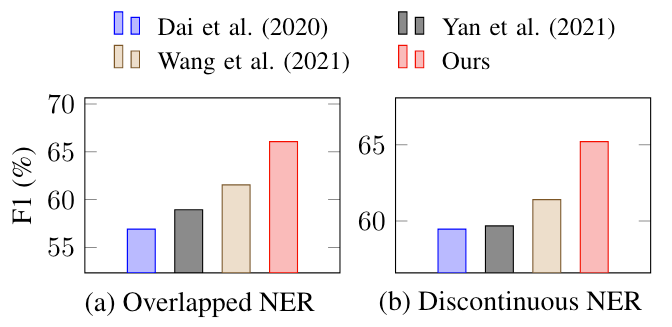### Model Ablation Studies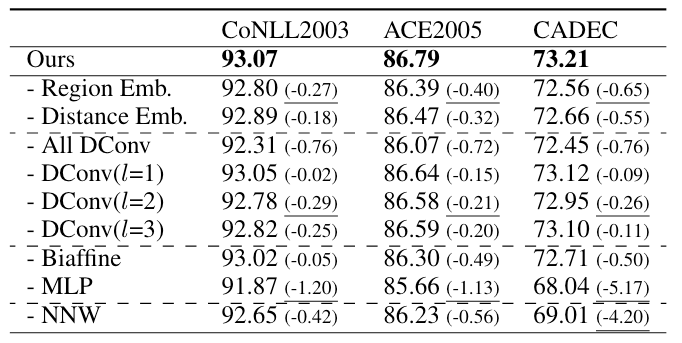MLP是挺猛的… 我倒是挺好奇CLN在Convolution Layer里面的作用, 可惜作者没有做实验.

## Summary

W2NER构建出一张Word Pair之间的二维表, 将现有的三大类NER任务转化成单词间关系分类问题, 因此可以统一到同一个NER框架当中.

上一篇OneEE: A One-Stage Framework for Fast Overlapping and Nested Event Extraction
OneEE: A One-Stage Framework for Fast Overlapping and Nested Event Extraction本文是论文OneEE: A One-Stage Framework for Fast
2022-11-21UniRE: A Unified Label Space for Entity Relation Extraction
UniRE: A Unified Label Space for Entity Relation Extraction本文是论文UniRE: A Unified Label Space for Entity Relation Extract
2022-09-22
目录